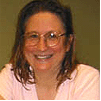top of page

# Math 5

Math 5

Thursday, 6:00-7:30

Teacher: Mary Marvin

Homework Hour:  Mondays from 7:00-8:00 p. m

Assistant Teacher: Collin Ostertag

Start date: 01/09/2023

No classes on Memorial weekend. End on June 18, 2023

Total #23 weeks. Tuition is paid in two installments. The first installment is paid for classes from Jan 7th to  April 10th, 2023

Curriculum

We continue with fractions, multiplying and dividing fractions with different denominators; more complicated fraction word problems will be studied. Students will move to percentages, ratios, rates, and geometry topics including the area of a triangle and composite figures, angles, and parallel lines. Along with the rigorous arithmetic program, Olympiad and contest problems and topics will be included as a part of each lesson.  Counting principles, finding probabilities, set theory, combinatorics and logic are some of the topics we will study for the Olympiads.

Prerequisites

Students must have mastery of 2-digit by 2-digit multiplication, division of a 4-digit number by a 1-digit number, concepts of fractions including addition and subtraction with different denominators, and fraction multiplication of a whole number by a fraction.  They must be able to work through a fraction word problem. Students must understand factors and multiples. Decimals must be mastered through addition, subtraction, multiplication, and division of a decimal number by a whole number, and rounding.  They must know how to find the area of a composite figure composed of squares and rectangles.

## Back to Schedule